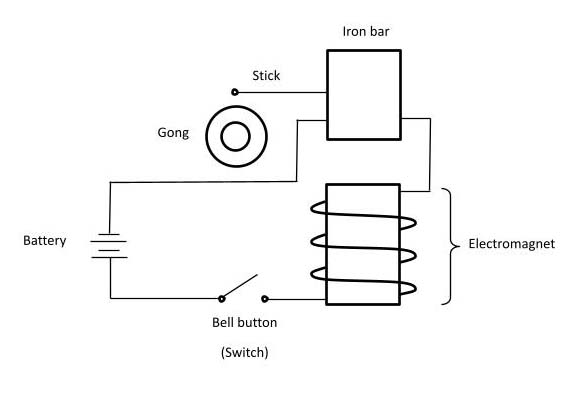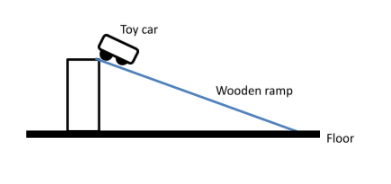# How to successfully grasp the topics of Energy and Forces in PSLE Science?

## 28 JanHow to successfully grasp the topics of Energy and Forces in PSLE Science?Energy and Forces are the first two topics that students will encounter when they begin their Primary 6 science classes.Energy
P6 students would have encountered different types of energies from both Upper and Lower Primary topics. This P6 topic will further introduce two new types of energy, Kinetic Energy and Potential Energy. Furthermore, this topic will introduce the concept of energy conversion, of which practically all energy questions in PSLE and School Examinations will allude to.
Many students (and parents) will be surprised that these aforementioned questions will not only include content from this topic, but will require the student to have mastery of several previous topics, namely heat, light and electricity. Many students will require a new set of skills to generate the answer on the concepts of a few topics in one question.

Forces
The second topic which most P6 students will encounter will be forces. Students are expected to remember different types of forces, their properties and their effects under different conditions.
Many students have to analyse which concepts and key points to be used for each and every question.How to Master both Energy and Forces

Though calculations of the exact amount of energy and strength of forces are not required for PSLE, students are expected to know how different factors will affect different types of energy and forces. For example, gravitational potential energy increases when either mass or height from the ground increases, whereas gravitational force only increases when the weight increases. Since questions can very easily include both forces and energy, students must be able to differentiate between energy and forces without mixing them up!

Furthermore, since forces and energy are actually quantifiable quantities, questions do regularly present data in both graphs and tables. Students must be able to interpret the data from tables and graphs, and draw relationships, trends and conclusion from them. For example, questions based on graphs displaying the change in gravitational potential and kinetic energies of a pendulum while it is oscillating is extremely common, and students may even be tasked to predict or plot new graphs when changes to the pendulum are made.

Of course, it goes without saying that constant practice from assessment books and school exam papers are essential to increase exposure to a myriad of energy and forces questions and train the student up in applying their knowledge and skills to answer successfully.

Check out the examples below on the common answers provided by the students and how it affects the accuracy and quality.  Contact us to make enquiries on our PSLE Science Achievers’ Program.  Your child’s achievements are our motivation!

MCQ Example

Study the set-up belowWhich is the energy change when the bell button is pressed?

1. Electrical energy –> kinetic energy –> sound energy + heat energy
2. Chemical energy –> electrical energy –> kinetic energy –> sound energy + heat energy
3. Chemical energy –> electrical energy –> kinetic energy –> sound energy
4. Chemical energy –> kinetic energy –> electrical energy + sound energy + heat energy

Comments: Most students will choose either option 1 or 3 which are wrong.

They have the misconceptions that battery produces electrical energy when it is connected to a circuit. In fact, all batteries contain stored chemical energy which is then converted to electrical energy after connecting them to a circuit.  Thus, option 1 is wrong.
Another common flaw is that most students neglect the fact that when the stick strikes the bell, friction occurs since both objects rub against each other.  This in turn gives rise to the production of heat other than sound.  Thus, option 3 is wrong.

Explanation: When the switch is closed, the circuit is connected.  The chemical energy stored in the battery will be converted to electrical energy.  This magnetises the electromagnet which attracts the iron bar.  In the process, the stick will move and strike the gong, producing sound and heat.  Thus, electrical energy is converted to kinetic energy which is then converted to sound energy and heat energy.

Structured question example

Raymond set up a ramp as shown in the diagram. A toy car was released from the top of the wooden ramp. The time taken for the toy car to reach the floor was recorded. The experiment was carried out again by replacing the wooden ramp with a plastic ramp. The table below shows the results of the experiment.Ramp Time taken (second) Wooden 3.4 Plastic 2.5

a) What is the force that causes the toy car to roll down the ramp? (1m)

a) Push [wrong]

Comment: “Push” is not the precise answer with respect to the context of the question.

a) Gravitational potential energy [wrong]

Comment: “Gravitational potential energy” is not relevant to the question as it is asking for force, not energy.

a) Gravitational force [correct]

Comment: “Gravitational force” is the pulling force of gravity.  It is also known as the weight which we measure the amount of gravitational force required to pull the toy car (down the ramp) towards the Earth.  Thus, this is the precise answer which fits well into the context of the question.

b) Compare the time taken for the toy car to reach the floor as shown in the table. (1m)

b) The toy car took 3.4s to roll down the wooden ramp to reach the floor but it took 2.5s to reach the floor on a plastic ramp. [wrong]

Comment: This answer does not examine the difference between the 2 values of time taken.  No comparison is made.

b) The toy car took a shorter time to reach the floor on a plastic ramp than on a wooden ramp. [correct]

Comment: By mentioning shorter time which is the correct keyword, a clear comparison is made in the time taken for the toy car.

c) Give a possible reason why this happened. (1m)

c) The plastic ramp is smooth, so the time taken is shorter. [wrong]

Comment: This answer does not consist of the explanation in terms of forces and specific keywords are missing.

c) The plastic ramp is smooth, so there is less friction and the time taken is shorter. [partially correct]

Comment: This answer does not specify the friction between which surfaces.  Further clarity is required.

c) As the plastic ramp is smoother than the wooden ramp, there is lesser friction between the toy car and the plastic ramp.  Thus, the time taken will be shorter. [correct]

Comment: This answer explains clearly the concept of frictional force and how it affects the time taken for the toy car to reach the floor.  When the surface is smoother, there is less friction between the plastic ramp and the toy car.  More gravitational force can be used to pull the toy car down the ramp towards the floor (Earth) instead of overcoming the frictional force.  Therefore, the time taken is shorter than the wooden ramp.

Einstein Takeaway

At Einstein, effective Science answering skills are imparted to students.  We train the students up to think more in-depth using the correct keywords.  We develop their answering techniques by crafting out the relevant concepts and specific keywords in clear written sentences.  They will be taught to identify the keywords and avoid the common errors so as to achieve a higher score especially in the structured questions during the exam and PSLE.  Contact us to make enquiries on our PSLE Science Achievers’ Program.  Your child’s achievements are our motivation!